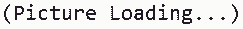You've successfully subscribed to The Finbox Blog
Welcome back! You've successfully signed in.# Time Value of Money Explained: Formula, Examples, And More

### What Is The Time Value of Money?

By definition, the time value of money is a simple concept that money available in the present is worth more than the same amount of money in the future. It can be easily explained with an example:

If you get paid \$2.000 for a job, you can invest the money, earn a 10% interest rate, and have \$200 more in your bank account after one (1) year. If your client pays you one (1) year late, you will lose \$200. That's why \$2.000 now is worth more than the same amount of money one year from now.

### Time Value vs. Purchasing Power

Inflation is an important factor when it comes to the time value of money because it tends to erode the purchasing power of money over time. For instance, the price of a gallon of gasoline today is more than doubled than twenty (20) years ago, which means you could have purchased a lot more gasoline for the same amount of money than you could today.Source: Statista

This is the main reason why both purchasing power and inflation needs to be factored in when you are thinking about investing your money. To calculate the real return on your investment, you have to subtract the inflation rate from the internal rate of return (IRR) of your investment. If the inflation rate is higher than the annualized investment return, you lose money even if you get a decent nominal return.

### Time Value of Money: Formula Explained

So, now that we’ve got a grip on the time value of money concept, let’s take a look at the formula that is used by individuals and businesses who want to make sure they have made the right investment decision. A formula that can be used for calculating the future value of money to compare it to the current value of money is:

FV = PV x [1 + (I / n)] (n x t)

Here's a breakdown of the individual components of the formula:

• FV: is the future value of money
• PV: is the current value of money
• I: is the interest rate that could be earned
• T: are the number of years it will take
• N: is the number of compounding periods of interest per year

### Time Value of Money: Example Calculations

The future value of \$50,000 invested for one year at 8% interest is:

FV = \$50.000 x [1 + (8% / 1)] ^ (1 x 1) = \$54.000

You can also find the net present value of a future amount. For example, how much did Alex invest one year ago if he gained an 8% interest rate and currently has \$10.000?

PV = \$10.000 / [1 + (8% / 1)] ^ (1 x 1) = \$9,259.26

If you want to dig deeper about net present value, I recommend reading this guide where we discuss everything you need to know about it.

Tags